﻿

### Diagonal operators, s-numbers and Bernstein pairs

#### Abstract

Replacing the nested sequence of "finite" dimensional subspaces by the nested sequence of "closed" subspaces in the classical Bernstein lethargy theorem, we obtain a version of this theorem for the space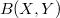$B (X,Y)$ of all bounded linear maps. Using this result and some properties of diagonal operators, we investigate conditions under which a suitable pair of Banach spaces form an exact Bernstein pair. We also show that many "classical" Banach spaces, including the couple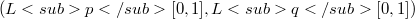$(Lp[0,1], Lq[0,1])$ form a Bernstein pair with respect to any sequence of s- numbers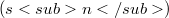$(sn)$, for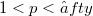$1< p < ∈fty$ and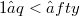$1≤ q < ∈fty$.

DOI Code: 10.1285/i15900932v17p209

Full Text: PDF